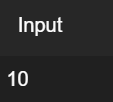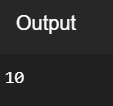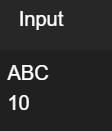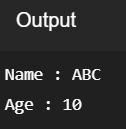# Input in C++

• Difficulty Level : Basic
• Last Updated : 01 Feb, 2021

The cin object in C++ is used to accept the input from the standard input device i.e., keyboard. it is the instance of the class istream. It is associated with the standard C input stream stdin. The extraction operator(>>) is used along with the object cin for reading inputs. The extraction operator extracts the data from the object cin which is entered using the keyboard.

Program 1:

Below is the C++ program to implement cin object to take input from the user:

## C++

 `// C++ program to demonstrate the``// cin object``#include ``using` `namespace` `std;`` ` `// Driver Code``int` `main()``{``    ``int` `i;`` ` `    ``// Take input using cin``    ``cin >> i;`` ` `    ``// Print output``    ``cout << i;`` ` `    ``return` `0;``}`

Input:Output:Note: Multiple inputs can also be taken using the extraction operators(>>) with cin.

Program 2:

Below is the C++ program to implement multiple inputs from the user:

## C++

 `// C++ program to demonstrate the taking``// multiple inputs from the user``#include ``using` `namespace` `std;`` ` `// Driver Code``int` `main()``{``    ``string name;``    ``int` `age;`` ` `    ``// Take multiple input using cin``    ``cin >> name >> age;`` ` `    ``// Print output``    ``cout << ``"Name : "` `<< name << endl;``    ``cout << ``"Age : "` `<< age << endl;`` ` `    ``return` `0;``}`

Input:Output:My Personal Notes arrow_drop_up